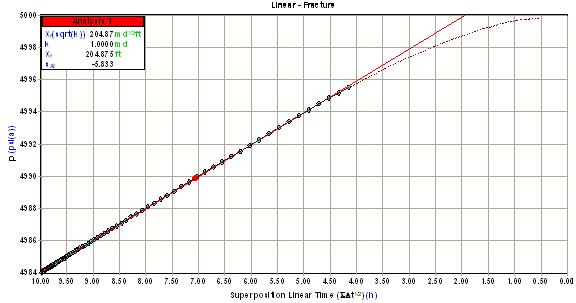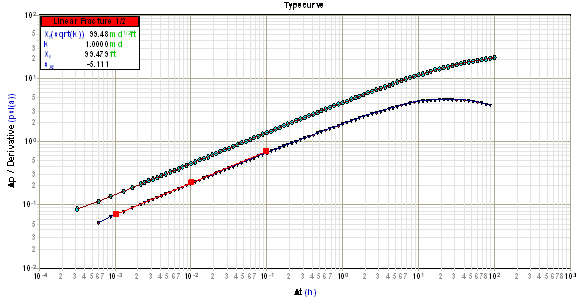# Linear Fracture Flow Analysis

Subtopics:

Linear fracture flow is one of the flow regimes that can exist when a well has been hydraulically fractured. The purpose of analyzing linear fracture flow data is to determine the fracture half-length (Xf).

## Constant Rate Solution (Field Units)

For oil and water:For gas:Linear fracture flow data will form a straight line when placed on a semi-log plot of pressure versus a square root time function (p vs. sqrt (time function)) for a buildup as shown below.The slope (m) of this line is used to calculate the product of the fracture half-length and the square root of permeability (Xf sqrt(k)) as follows:

For oil and water:For gas:The permeability can be obtained from the radial flow analysis, or estimated from core data, or other tests. After permeability is determined, fracture half-length is found using the following equation:## Summary of Equations for Linear Fracture Flow (Field Units)

Flow Period Fracture Half-Length * Square Root of Permeability (ft md0.5)

Oil

DrawdownBuildupGas

DrawdownBuildup## Other Required Equations (Field Units)

 SkinFor infinite conductivity fractures, when kf is large (FCD > 20)

## Derivative (Field Units)

The signature of linear fracture flow data on a derivative plot is a straight line with a slope of 1/2. The position of this line is used to calculate the product of fracture half-length and root permeability (Xf sqrt(k)). Note that the product of fracture half-length and root permeability increases as the position of this line moves to the right.

Starting with the constant rate solution (see above), the derivative with respect to the logarithm of square-root time is:

For oil and water:For gas:Taking the logarithm of both sides:

For oil and water:For gas:This result is linear with time and, as a result, the derivative of linear fracture flow data falls on a straight line with a slope equal to 1/2 on a log Dp versus log Dt plot as shown below.Using any derivative point on this line, the product of fracture half-length and root permeability (Xfsqrt(k)). can be determined as follows:

For oil and water:For gas:## Summary of Equations for Linear Fracture Flow Derivative Analysis (Field Units)

Flow Period Fracture Half-Length * Root Permeability (ft md0.5)

Oil

DrawdownBuildupGas

DrawdownBuildup